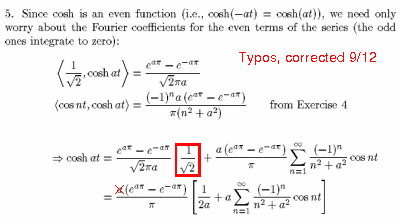Fall 2003

# Mathematics Math21b Fall 2003

## Linear Algebra and Differential Equations

Office: SciCtr 434
Email: knill@math.harvard.eduNew Syllabus Calendar Homework Challenge Exam Handout Check Exhibit Cas Faq Link

 Send questions of potential general interest to maths21a@fas.harvard.edu.
 Question: In Section 10.3, problem 6 uses problem 5 which we did not do. Any hint? Answer: Here is the solution to problem 5:Question: What exactly is this Jordan Normal form? Answer: Izzet has written down something about it.
 Question: Why don't we just use the formula P=AAT to find the matrix P of the orthogonal projection onto a subspace V? Answer: the formula P=A AT gives the orthogonal projection if the column vectors of A are orthonormal vectors only. In general one has to use a formula which was derived in least square solutions: P= A (AT A)-1 AT which simplifies in the case of orthonormal columns to A AT because the middle part is then an identity matrix.
 Question: Is there any difference between a space and a linear space and between a linear space and a subspace, and between a space and a subspace. Answer: Sometimes, space is used as an abbreviation for the three dimensional linear space. If a linear space is a subset of an other linear space, one calls it a "linear subspace". But it is still a linear space. Since any linear space is also a subspace of itself, it is also a "linear subspace". The additional "sub" can be used to stress that something is contained in something else.
 Question: How come that two rotations don't commute, but two rotation-dilations do? Answer: The key is the dimension. Rotations in the plane commute, while rotations in space do not. Also, two rotation-dilations commute in the plane, while they do not commute in space.
 Question: Are starred, "recommended" problems meant to be optional enrichment exercises or exercises to be turned in with the assignment? I was planning on doing them on my own, but not turning them in with the assignment. Answer: Recommended problems don't have to be turned in and CA's don't have to grade them. We plan to post solutions to the recommended problems and recommend to solve those problems if time permits. Otherwise, focus on the main problems. Don't feel pressured to do them. You can get perfect scores without doing them. You can use them also as practice problems for the exams.
 Question: When do classes start and what book will we used? Answer: Check out the syllabus page.
 Question: When will I get my section assignment? Answer: You should be notified by the program latest until Friday night. If you should not have got the section assignment until then, contact the course head Oliver Knill (knill@math).